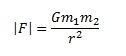# The Gravitational Field

## Homework Statement## Homework Equations## The Attempt at a SolutionBut as you can see, when I typed the answer on the answer column, the computer marked it as wrong. Please point out my mistake in 24 hours. Thank you.

Last edited:

lewando
Homework Helper
Gold Member
What is the distance between M and O?

What is the distance between M and O?

By simple Pythagorean theorem you can work it out to l^2 sqrt{2}

lewando
Homework Helper
Gold Member
By simple Pythagorean theorem you can work it out to l^2 sqrt{2}
I get something different. Since the clock is ticking, how about
$$l \sqrt{2}$$

I get something different. Since the clock is ticking, how about
$$l \sqrt{2}$$

I was writing the distance squared. So basically our answer for that is same.

lewando
Homework Helper
Gold Member
Ahh.. nevermind me, I thought I saw it... standby please.

Ahh.. nevermind me, I thought I saw it... standby please.

I think my answer is correct and the computer is wrong.

lewando
Homework Helper
Gold Member
How about your last step? The answer you gave was the common coefficient of i and j. But to get the magnitude should you not take the root of sum of the squares of these coefficients?

Last edited:
Use more sets of parentheses at the beginning.

How about your last step? The answer you gave was the common coefficient of i and j. But to get the magnitude should you not take the root of sum of the squares of these coefficients?

Enlighten me.

Hootenanny
Staff Emeritus
Gold Member
Enlighten me.
What is the magnitude of the vector $\boldsymbol{x} = \boldsymbol{i}+\boldsymbol{j} = [1,1]^\text{T}$?

When something is in the form Constant * ( i + j ) and you would like to find the magnitude of that

use abs ( Constant * ( i+j) ) = Abs(Constant ) * Abs(i+j)

what is Abs ( i + j ) ?

How would you interpret it ?